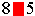SEARCH HOMEMath Central Quandaries & QueriesQuestion from marcha: when this three digit number is rounded to the nearest ten, the sum of its digits is 16 the hundreds digit of this number is 8 and the ones digit is 5, what is the number?Marcha,

Talking about "rounding to the nearest ten" is misleading, because (say) 20 and 30 are exactly the same distance from 25; there isn't a "nearest" ten. There are various conventions on how to "round fives". Schools usually teach that numbers ending in 5 should be rounded upward. (*)

Whatever: this won't change the hundreds digit.

So the number iswhere there is a missing digit in the red box

Now what do you get after rounding?

So what's in the red box?

Good Hunting!
-RD

* There is another convention called "round-to-even" or "banker's rounding"; you can look it up if you're curious.Math Central is supported by the University of Regina and The Pacific Institute for the Mathematical Sciences.